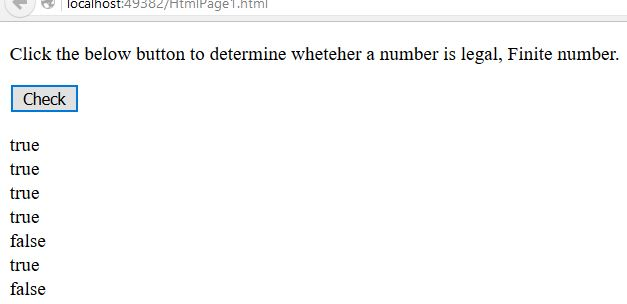# Check Finite, legal Number in JavaScript

##### How to check whether a number is a finite, legal number in JavaScript?

By using isFinite() function we can check whether a number is a finite, legal number. Finite number is a number that could be measured or given a value.

```<p>Click the below button to determine wheteher a number is legal, Finite number.</p>
<input type="button" onclick="mySearch()" value="Check">
<p id="myId"></p>

<script>
function mySearch() {
var p = isFinite(258) + "</br>";
var q = isFinite(25.8) + "</br>";
var r = isFinite(2 * 5 * 8) + "</br>";
var s = isFinite("258") + "</br>";
var t = isFinite("Sheo Narayan") + "</br>"; //* This is Infinite *//
var u = isFinite(0) + "</br>";
var v = isFinite("09-Nov-2015"); //* This is Infinite *//

var a = p + q + r + s + t + u + v;
document.getElementById("myId").innerHTML = A;
}
</script>
```

In the above code snippet we have given Id as "myId"to the second `<p>` element in the HTML code. There is a function mySearch() in the script code which is connected to the onclick of the HTML button.

We need to check whether a number is a finite, legal number. For that we are using isFinite() function, if the input value of isFinite() function is finite, legal number it returns the output as 'true' otherwise it returns 'false'.

Onclick of the button "Check" in the HTML code fires the function mySearch() in the script code at the same time isFinite() function check whether a number is a finite, legal number and returns the output.

OUTPUTViews: 2740 | Post Order: 181